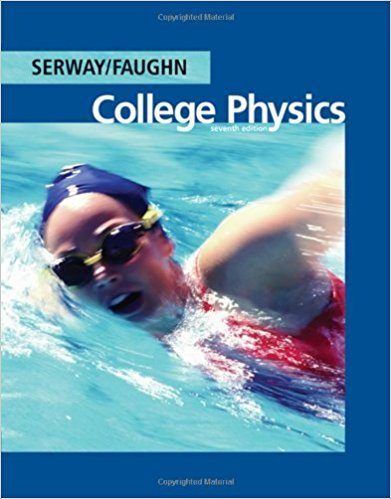×
Get Full Access to College Physics - 7 Edition - Chapter 14 - Problem 14.32
Get Full Access to College Physics - 7 Edition - Chapter 14 - Problem 14.32

×

# Two loudspeakers are placed above and below one another,ISBN: 9780495113690 154

## Solution for problem 14.32 Chapter 14

College Physics | 7th Edition

• Textbook Solutions
• 2901 Step-by-step solutions solved by professors and subject experts
• Get 24/7 help from StudySoup virtual teaching assistantsCollege Physics | 7th Edition

4 5 1 422 Reviews
31
3
Problem 14.32

Two loudspeakers are placed above and below one another, as in Figure 14.15, and are driven by the same source at a frequency of 500 Hz. (a) What minimum distance should the top speaker be moved back in order to create destructive interference between the speakers? (b) If the top speaker is moved back twice the distance calculated in part (a), will there be constructive or destructive interference?

Step-by-Step Solution:
Step 1 of 3

• Graphs o Velocityvs.TimeGraph § Straighthorizontallinemeans“constantvelocity” v t § Straightslopedlinemeans“constantacceleration”(accelerationisa changeinvelocity) v t o Positionvs.TimeGraph § Straighthorizontallinemeans“atrest” x

Step 2 of 3

Step 3 of 3

##### ISBN: 9780495113690

The answer to “Two loudspeakers are placed above and below one another, as in Figure 14.15, and are driven by the same source at a frequency of 500 Hz. (a) What minimum distance should the top speaker be moved back in order to create destructive interference between the speakers? (b) If the top speaker is moved back twice the distance calculated in part (a), will there be constructive or destructive interference?” is broken down into a number of easy to follow steps, and 68 words. College Physics was written by and is associated to the ISBN: 9780495113690. This textbook survival guide was created for the textbook: College Physics, edition: 7. Since the solution to 14.32 from 14 chapter was answered, more than 475 students have viewed the full step-by-step answer. The full step-by-step solution to problem: 14.32 from chapter: 14 was answered by , our top Physics solution expert on 11/15/17, 04:28PM. This full solution covers the following key subjects: back, top, speaker, moved, interference. This expansive textbook survival guide covers 30 chapters, and 2135 solutions.

Unlock Textbook Solution